# Assignments

Calculate a maximum, a minimum or a horizontal inflection point of the functions below. We restrict ourselves to local extremes.

1. Determine the extreme of the parabola: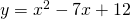Solution

2. Verify whether the graph of the function has extremes: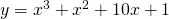and if so, whether it is a maximum of a minimum.

Solution

3. Determine the extreme of the function: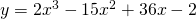and verify whether it is a maximum or a minimum using the sign of the first derivative.

Solution

4. Determine the extreme of the function:and verify whether it is a maximum or a minimum by using the second derivative.

Solution

5. Find the extremes of the function: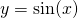on the interval interval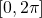.

Solution

6. Find the extremes of the function: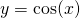on the interval. Verify whether they are maxima or minima by using the second derivative.

Solution

7. Determine the extremes of the function: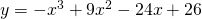Verify in which point there is a maximum or a minimum by using the second derivative.

Solution

8. Verify whether the function: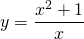has a maximum or a minimum.

Solution

9. Verify whether the function: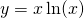has a maximum or a minimum.

Solution

10. Find the extreme of the function:without using the sign of the first derivative or the second derivative.

Solution

0